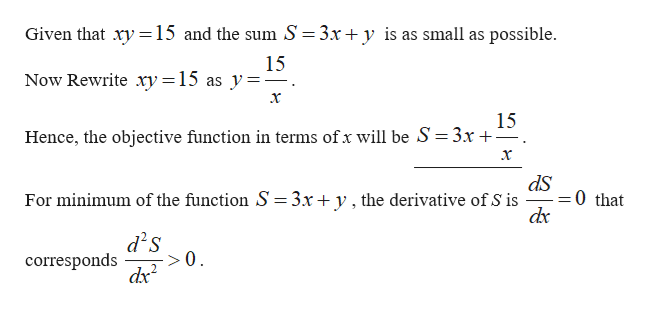# Find positive numbers x and y satisfying the equation xy=15 such that the sum 3x+y is as small as possible.Let S be the sum of the two numbers. What is the objective function in terms of one number, x?S=?(type an expression)The numbers are x=? and y=? (type exact numbers, using radicals as needed)

Question
51 views

Find positive numbers x and y satisfying the equation xy=15 such that the sum 3x+y is as small as possible.

Let S be the sum of the two numbers. What is the objective function in terms of one number, x?

S=?(type an expression)

The numbers are x=? and y=? (type exact numbers, using radicals as needed)

check_circle

Step 1help_outlineImage TranscriptioncloseGiven that xy15 and the sum S = 3x + y is as small as possible 15 Now Rewrite xy = 15 as y= x 15 Hence, the objective function in terms of x will be S 3x +- x 0 that For minimum of the function S =3x+ y, the derivative of S is dx d s corresponds >0 fullscreen

### Want to see the full answer?

See Solution

#### Want to see this answer and more?

Solutions are written by subject experts who are available 24/7. Questions are typically answered within 1 hour.*

See Solution
*Response times may vary by subject and question.
Tagged in